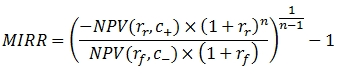# SQL Server 2005 modified internal rate of return function

MIRR_q

Updated: 5 August 2010
Use MIRR_q to calculate the modified internal rate of return, where positive and negative cash flows are financed at different rates

Given a vector of cash flows, the equation for MIRR is:Where
n = Number of cashflows
c+ = The greater of 0 or the cash flow amount
c- = The lesser of 0 or the cash flow amount
rr = Reinvestment rate
rf = Finance rate
NPV = Net Present Value function
Syntax
SELECT [westclintech].[wct].[MIRR_q] (
<@Cashflows_RangeQuery, nvarchar(4000),>
,<@Finance_rate, float,>
,<@Reinvest_rate, float,>)

Arguments
@Cashflows_RangeQuery
a select statement, as text, which specifies the cash flow values to be used in the modified internal rate of return calculation.
@Finance_rate
the rate to be applied to negative cash flows. @Finance_rate is an expression of type float or of a type that can be implicitly converted to float.

@Reinvest_rate
the rate to be applied to positive cash flows. @Reinvest_rate is an expression of type float or of a type that can be implicitly converted to float.
Return Type
float
Remarks
·         This function requires that there be at least one positive cash flow and one negative cash flow.
·         The formula for modified internal rate of return is:

MIRR = (((-NPV(@Reinvest_rate, reinvest_cash_flows) * (1 + @Reinvest_rate) ^ n) / (NPV(@Finance_rate, finance_cash_flows) * (1 + @Finance_rate))) ^ (1 / (n - 1))) - 1

Where n is the number cash flows.
Example

Create a table to store cash flow projections, by year, for a variety of projects:
CREATE TABLE [dbo].[cf1](
[proj_no] [float] NOT NULL,
[period] [float] NOT NULL,
[cf_amt] [float] NOT NULL,
CONSTRAINT [PK_cf1] PRIMARY KEY CLUSTERED
(
[proj_no] ASC,
[period] ASC
)WITH (PAD_INDEX = OFF, STATISTICS_NORECOMPUTE = OFF, IGNORE_DUP_KEY = OFF, ALLOW_ROW_LOCKS = ON, ALLOW_PAGE_LOCKS = ON) ON [PRIMARY]
) ON [PRIMARY]
Insert the data for 3 projects into the table.
INSERT INTO cf1 VALUES(1,1,-25000)
INSERT INTO cf1 VALUES(1,2,5000)
INSERT INTO cf1 VALUES(1,3,10000)
INSERT INTO cf1 VALUES(1,4,15000)
INSERT INTO cf1 VALUES(1,5,20000)
INSERT INTO cf1 VALUES(1,6,25000)
INSERT INTO cf1 VALUES(2,1,-25000)
INSERT INTO cf1 VALUES(2,2,25000)
INSERT INTO cf1 VALUES(2,3,10000)
INSERT INTO cf1 VALUES(2,4,15000)
INSERT INTO cf1 VALUES(2,5,10000)
INSERT INTO cf1 VALUES(2,6,5000)
INSERT INTO cf1 VALUES(3,1,-25000)
INSERT INTO cf1 VALUES(3,2,5000)
INSERT INTO cf1 VALUES(3,3,25000)
INSERT INTO cf1 VALUES(3,4,10000)
INSERT INTO cf1 VALUES(3,5,20000)
INSERT INTO cf1 VALUES(3,6,15000)

Enter a SELECT statement to calculate the MIRR for the 3 projects so as to compare the results, assuming a 10% finance rate and a 12% reinvestment rate:
select d.proj_no
,wct.MIRR_q(
'SELECT c.cf_amt
from cf1 c
where c.proj_no = ' + convert(char, d.proj_no)
,.10
,.12) as MIRR
from cf1 d
group by d.proj_no
Here is the result set
proj_no                MIRR
---------------------- ----------------------
1                      0.286588269972987
2                      0.287376740493951
3                      0.300312295003069

### SupportCopyright 2008-2021 Westclintech LLC         Privacy Policy        Terms of Service